# Math: Middle School: Grades 6, 7 and 8 Quiz - Shapes - Angles 2 (Questions)

This Math quiz is called 'Shapes - Angles 2' and it has been written by teachers to help you if you are studying the subject at middle school. Playing educational quizzes is a fabulous way to learn if you are in the 6th, 7th or 8th grade - aged 11 to 14.

It costs only \$12.50 per month to play this quiz and over 3,500 others that help you with your school work. You can subscribe on the page at Join Us

Can you tell an acute angle from an obtuse angle? Do you know how many degrees there are in a circle or in a triangle? Are you familiar with the angles of a regular pentagon or a regular hexagon?

Play this quiz to gain an acute knowledge of angles.

1. The angle in the picture below is a(n) .......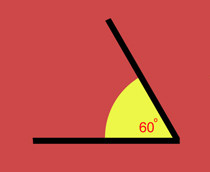[ ] acute angle [ ] obtuse angle [ ] reflex angle [ ] right angle
2. The angle in the picture below is a(n) .......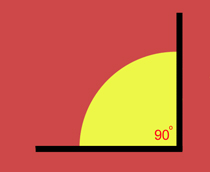[ ] acute angle [ ] obtuse angle [ ] reflex angle [ ] right angle
3. The angle in the picture below is a(n) .......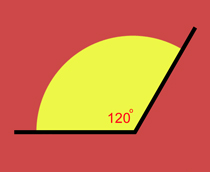[ ] acute angle [ ] obtuse angle [ ] reflex angle [ ] right angle
4. The angle in the picture below is a(n) .......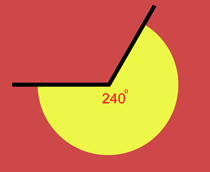[ ] acute angle [ ] obtuse angle [ ] reflex angle [ ] right angle
5. What is 'angle A' in the diagram below?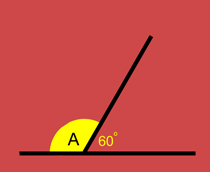[ ] 80o [ ] 100o [ ] 120o [ ] 140o
6. What is 'angle A' in the diagram below?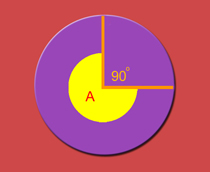[ ] 90o [ ] 180o [ ] 210o [ ] 270o
7. What is 'angle A' in the diagram below?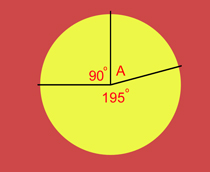[ ] 55o [ ] 65o [ ] 75o [ ] 85o
8. What is 'angle A' in the equilateral triangle below?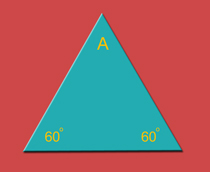[ ] 50o [ ] 60o [ ] 70o [ ] 80o
9. What is 'angle A' in the regular pentagon below?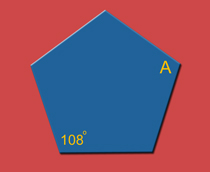[ ] 80o [ ] 88o [ ] 92o [ ] 108o
10. What is 'angle A' in the regular hexagon below?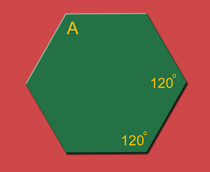[ ] 80o [ ] 120o [ ] 180o [ ] 240oMath: Middle School: Grades 6, 7 and 8 Quiz - Shapes - Angles 2 (Answers)
1. The angle in the picture below is a(n) .......[x] acute angle [ ] obtuse angle [ ] reflex angle [ ] right angle
Acute angles are less than 90o
2. The angle in the picture below is a(n) .......[ ] acute angle [ ] obtuse angle [ ] reflex angle [x] right angle
Right angles are exactly 90o
3. The angle in the picture below is a(n) .......[ ] acute angle [x] obtuse angle [ ] reflex angle [ ] right angle
Obtuse angles are between 90o and 180o
4. The angle in the picture below is a(n) .......[ ] acute angle [ ] obtuse angle [x] reflex angle [ ] right angle
Reflex angles are between 180o and 360o
5. What is 'angle A' in the diagram below?[ ] 80o [ ] 100o [x] 120o [ ] 140o
The angles on a straight line add up to 180o
6. What is 'angle A' in the diagram below?[ ] 90o [ ] 180o [ ] 210o [x] 270o
The angles in a circle add up to 360o
7. What is 'angle A' in the diagram below?[ ] 55o [ ] 65o [x] 75o [ ] 85o
90o + 195o + 75o = 360o
8. What is 'angle A' in the equilateral triangle below?[ ] 50o [x] 60o [ ] 70o [ ] 80o
The angles in a triangle add up to 180o
9. What is 'angle A' in the regular pentagon below?[ ] 80o [ ] 88o [ ] 92o [x] 108o
All angles in a regular pentagon are the same. (The length of the sides are also the same)
10. What is 'angle A' in the regular hexagon below?[ ] 80o [x] 120o [ ] 180o [ ] 240o
Remember that all the angles in a regular hexagon are the same and all the lengths of the sides are the same